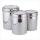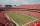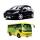# Sum of three numbers

The sum of three numbers from which second number is 20% smaller than the first number and the third number is 25% smaller than the second number is 96. Determine this numbers.

Result

x =  40
y =  32
z =  24

#### Solution:

x+y+z=96
y=x-0.20x
z=y-0.25y

x+y+z = 96
0.8x-y = 0
0.75y-z = 0

x = 40
y = 32
z = 24

Calculated by our linear equations calculator.

Leave us a comment of example and its solution (i.e. if it is still somewhat unclear...):Be the first to comment!#### To solve this example are needed these knowledge from mathematics:

Our percentage calculator will help you quickly calculate various typical tasks with percentages. Do you have a linear equation or system of equations and looking for its solution? Or do you have quadratic equation?

## Next similar examples:

1. Two numbersWe have two numbers. Their sum is 140. One-fifth of the first number is equal to half the second number. Determine those unknown numbers.
2. The largerThe larger of two numbers is nine more than four times the smaller number. The sum of the two numbers is fifty-nine. Find the two numbers.
3. ContainerFirst day got off 1/4 water from full container, second day 1/5 of the remaining water. How many percent remain container filled?
4. Unknown numberIdentify unknown number which 1/5 is 40 greater than one tenth of that number.
5. TVsProduction of television sets increased from 3,500 units to 4,200 units. Calculate the percentage of production increase.Number 118 divide into two addends, so first addend is 69 greater than 75% of the second addend.
7. PercentsHow many percents is 900 greater than the number 750?
8. Base, percents, valueBase is 344084 which is 100 %. How many percent is 384177?
9. Tickets1260 tickets sold. On the first day, 80% was sold on the second day was sold. How many tickets were sold first and how much the next day?
10. TheatroTheatrical performance was attended by 480 spectators. Women were in the audience 40 more than men and children 60 less than half of adult spectators. How many men, women and children attended a theater performance?
11. TicketsOn the football tournament ticket cost 45 Kc for standing and 120kč for sitting. Sitting spectators was 1/3 more than standing. The organizers collected a total 12 300 Kc. How many seated and standing seats (spectators)?
12. Two trainsThere were 159 freight wagons on the railway station creating 2 trains. One had 15 more wagons than the other. How many wagons did each train have?
13. Parking120 vehicles parking on the morning. Pasenger car is charged 20 CZK, 50 CZK per bus. The guard collected for parking 2640 CZK in total. How many cars and how many buses stood in the parking?
14. ATC campThe owner of the campsite offers 79 places in 22 cabins. How many of them are triple and quadruple?
15. The ballThe ball was discounted by 10 percent and then again by 30 percent. How many percent of the original price is now?
16. PersonsPersons surveyed:100 with result: Volleyball=15% Baseball=9% Sepak Takraw=8% Pingpong=8% Basketball=60% Find the average how many like Basketball and Volleyball. Please show your solution.
17. Rabbits 3Viju has 40 chickens and rabbits. If in all there are 90 legs. How many rabbits are there with Viju?